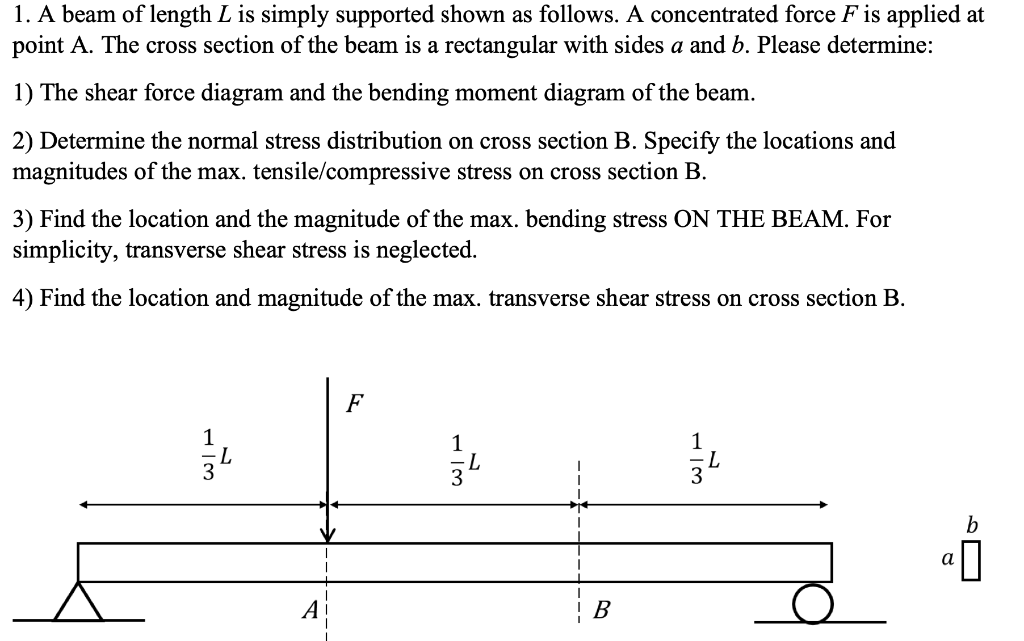Home / Expert Answers / Mechanical Engineering / 1-a-beam-of-length-l-is-simply-supported-shown-as-follows-a-concentrated-force-f-is-pa897

# (Solved): 1. A beam of length $$L$$ is simply supported shown as follows. A concentrated force $$F$$ is ...1. A beam of length $$L$$ is simply supported shown as follows. A concentrated force $$F$$ is applied at point $$\mathrm{A}$$. The cross section of the beam is a rectangular with sides $$a$$ and $$b$$. Please determine: 1) The shear force diagram and the bending moment diagram of the beam. 2) Determine the normal stress distribution on cross section B. Specify the locations and magnitudes of the max. tensile/compressive stress on cross section B. 3) Find the location and the magnitude of the max. bending stress ON THE BEAM. For simplicity, transverse shear stress is neglected. 4) Find the location and magnitude of the max. transverse shear stress on cross section B.

We have an Answer from Expert